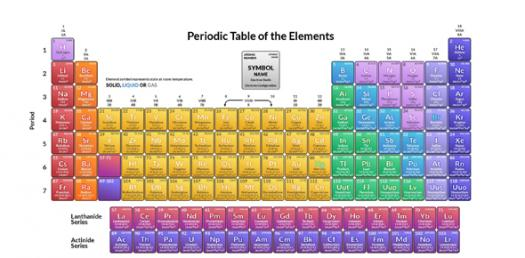# Chapter 5 - Periodic Table

19 Questions | Total Attempts: 103SettingsThe periodic table can be used to predict properties of elements, even those that are yet to be discovered. By studying it you get to understand some trends in a group of elements and how chemical reactions may occur. Below is a quiz to test you on Chapter 5 which covered the organization, properties, and discovery of the periodic table.

• 1.
The shorthand electron configuration [Ar] 4s2 4d1 is that of the element scandium.
• A.

True

• B.

False

• 2.
A division of the periodic table is labeled with the number 14. This section can be called a family.
• A.

True

• B.

False

• 3.
The lanthanides and actinides are in group 3 of the periodic table.
• A.

True

• B.

False

• 4.
The noble gases are located in the d block of elements.
• A.

True

• B.

False

• 5.
Newlands left spaces in his periodic table and predicted the properties of the elements that belonged there.
• A.

True

• B.

False

• 6.
Doberiner's quartets stated that elements 2 and 3 have properties halfway between elements 1 and 4.
• A.

True

• B.

False

• 7.
Germanium has a higher metallic character then sodium.
• A.

True

• B.

False

• 8.
The most common ion for the element Aluminum (Al) has a larger ionic radii than the most common ion for the element bromide.
• A.

True

• B.

False

• 9.
Atomic radii is measured in the unit am.
• A.

True

• B.

False

• 10.
Period 6 elements have a lower ionization energy than period 2 elements.
• A.

True

• B.

False

• 11.
What is another name for a period?
• A.

Series

• B.

Series

• C.

SERIES

• 12.
How many groups make up the s block of elements?
• A.

2

• B.

Two

• C.

Two

• D.

TWO

• 13.
What is the main thing Mendeleev knowingly based the order of his periodic table on, causing him to order it like no one else had done before?
• A.

Atomic number

• B.

Chemical properties

• C.

Physical properties

• D.

Atomic mass

• 14.
What did Mosley use to determine the atomic number of the elements?
• A.

An electron microscope

• B.

An electron balance

• C.

A compilation of data from other scientists

• D.

X-Rays

• 15.
Which scientist discovered the group 18 elements, the noble gases?
• A.

Ramsey

• B.

Mayer

• C.

Mendeleev

• D.

Newlands

• 16.
The element with the configuration [Ar] 4s2 3d10 4p1 is located in which period and group?
• A.

Period 6 No Group

• B.

Period 4 Group 13

• C.

Period 2 Group 2

• D.

No period Group 14

• 17.
What can be defined as "The energy change that occurs when an atom gains an electron to form an ion?"
• A.

Ionization energy

• B.

• C.

Electron affinity

• D.

Metallic character

• 18.
• A.

It increase from left to right across a period

• B.

It increases as you go down a group

• C.

It is one-fourth the distance between the nuclei of identical bonded atoms

• D.

It decrease from left to right across a period

• 19.
The elements in which block(s) are known as the main group elements?
• A.

D and f

• B.

S and d

• C.

P and s

• D.

D only

Related TopicsBack to top